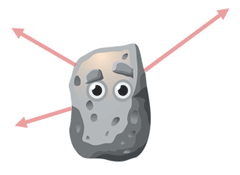Combining Vectors: Three Forces
View the Lesson | MATHguide homepage Updated March 27th, 2021

Problem:     Three forces are acting on a body. Determine the resultant vector that is acting on the body. Write the solution in component form, linear combinbation form, and as a magnitude and direction. Round your responses to the nearest tenth of a unit.

 F1 = 62 Newtons at 66° F2 = 69 Newtons at 3° F3 = 24 Newtons at 291°This is a generic diagram and doesnot reflect the given forces.

Solution:   component form → < , >

linear combination formi + j

magnitude Newtons
direction°